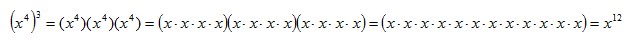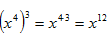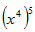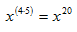Power of a Power

To simplify an expression that has an exponent raised to a power, multiply the existing exponent by the power.

Power of a Power Rule : (xn)m = xn·m

To see why this rule works, see the expansion below:Therefore,.

Example

Simplify.

Use the Power of a Power Rule to multiply the exponents.